## Thomson Scattering

The cross section for Thomson scattering illustrates the need for negative energy'' states in our calculations. Recall that we got the correct cross section from the non-relativistic calculation and that Thomson also got the correct result from classical E&M.

In the Dirac theory, we have only one term in the interaction Hamiltonian,Because it is linear in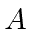it can create a photon or annihilate a photon. Photon scattering is therefore second order (and proportional to). The quantized photon field isThe initial and final states are definite momentum states, as are the intermediate electron states. We shall first do the calculation assuming no electrons from the negative energy'' sea participate, other than to exclude transitions to those negative energy'' states. The initial and final states are therefore the positive energy plane wave statesfor. The intermediate states must also be positive energy states since the negative energy'' states are all filled.

The computation of the scattering cross section follows the same steps made in the development of the Krammers-Heisenberg formula for photon scattering. There is noterm so we are just computing the two second order terms.As in the earlier calculation, the photon states have been eliminated from the equation since they give a factor of 1 with the initial state photon being annihilated and the final state photon being created in each term.

Now lets take a look at one of the matrix elements. Assume the initial state electron is at rest and that the photon momentum is small.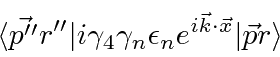For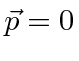and, a delta function requires that. It turns out that, so that the cross section is zero in this limit.This matrix only connectsspinors to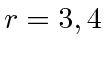spinors because of its off diagonal nature. So, the calculation yields zero for a cross section in contradiction to the other two calculations. In fact, since the photon momentum is not quite zero, there is a small contribution, but far too small.

The above calculation misses some important terms due to the negative energy'' sea. There are additional terms if we consider the possibility that the photon can elevate a negative energy'' electron to have positive energy.In one term, the initial state photon is absorbed by a negative energy'' electron, then the initial state electron fills the hole in the negative energy'' sea emitting the final state photon. In the other term, even further from the mass shell, a negative energy'' electron emits the final state photon and moves to a positive energy state, then the initial state electron absorbs the initial photon and fills the hole left behind in the sea. These terms are larger because the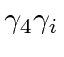matrix connects positive energy and negative energy'' states.The matrix element is to be taken with the initial electron at rest,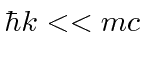, the final electron (approximately) at rest, and hence the intermediate electron at rest, due to a delta function of momentum conservation that comes out of the spatial integral.

Let the positive energy spinors be written as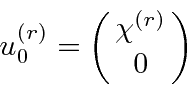and the negative energy'' spinors as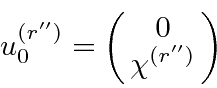The matrixconnect the positive and negative energy'' spinors so that the amplitude can be written in terms of two component spinors and Pauli matrices.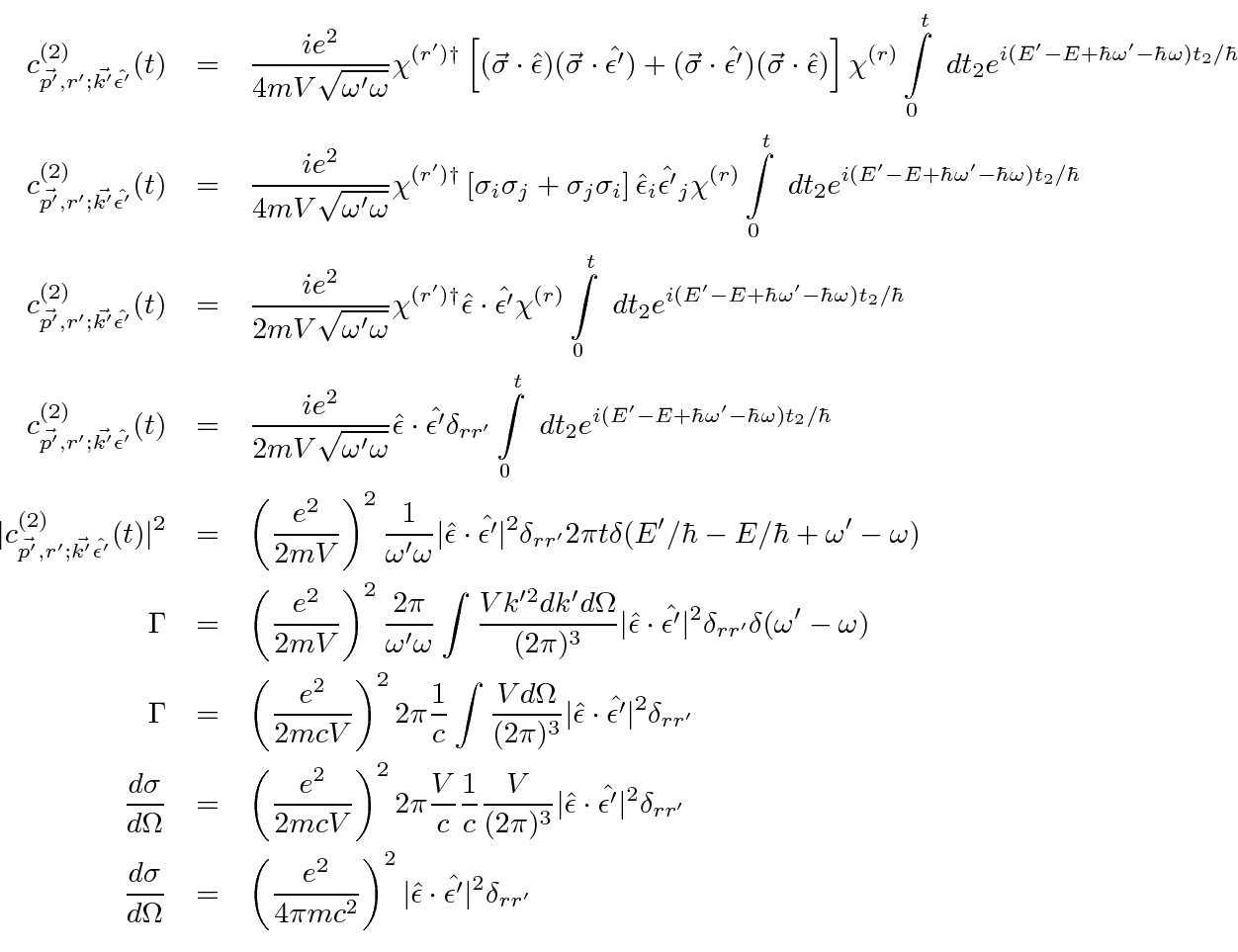This agrees with the other calculations and with experiment. The negative energy'' sea is required to get the right answer in Dirac theory. There are alternatives to the negative energy'' sea. Effectively we are allowing for the creation of electron positron pairs through the use of the filled negative energy states. The same result could be obtained with the possibility of pair creation, again with the hypothesis that a positron is a negative energy'' electron going backward in time.

Jim Branson 2013-04-22